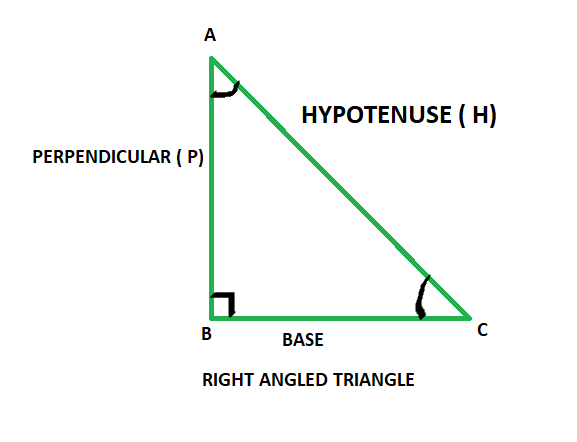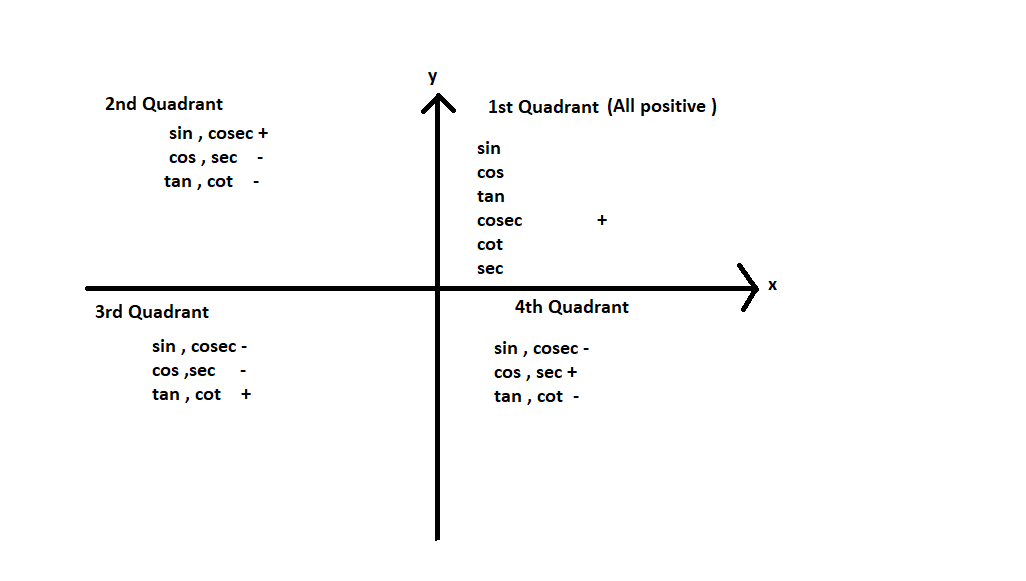# Find the exact value of csc 270°

• Last Updated : 12 Oct, 2021

Trigonometry is a discipline of mathematics that studies the relationships between the lengths of the sides and angles of a right-angled triangle. Trigonometric functions, also known as goniometric functions, angle functions, or circular functions, are functions that establish the relationship between an angle to the ratio of two of the sides of a right-angled triangle. The six main trigonometric functions are sine, cosine, tangent, cotangent, secant, or cosecant.

Angles defined by the ratios of trigonometric functions are known as trigonometry angles. Trigonometric angles represent trigonometric functions. The value of the angle can be anywhere between 0-360°.

Attention reader! All those who say programming isn't for kids, just haven't met the right mentors yet. Join the  Demo Class for First Step to Coding Coursespecifically designed for students of class 8 to 12.

The students will get to learn more about the world of programming in these free classes which will definitely help them in making a wise career choice in the future.As given in the above figure in a right-angled triangle:

• Hypotenuse: The side opposite to the right angle is the hypotenuse, It is the longest side in a right-angled triangle and opposite to the 90° angle.
• Base: The side on which angle C lies is known as the base.
• Perpendicular: It is the side opposite to angle C in consideration.

### Trigonometric Functions

Trigonometry has 6 basic trigonometric functions, they are sine, cosine, tangent, cosecant, secant, and cotangent. Now let’s look into the trigonometric functions. The six trigonometric functions are as follows,

• sine: It is defined as the ratio of perpendicular and hypotenuse and It is represented as sin θ
• cosine: It is defined as the ratio of base and hypotenuse and it is represented as cos θ
• tangent: It is defined as the ratio of sine and cosine of an angle. Thus the definition of tangent comes out to be the ratio of perpendicular and base and is represented as tan θ
• cosecant: It is the reciprocal of sin θ and is represented as cosec θ.
• secant: It is the reciprocal of cos θ and is represented as sec θ.
• cotangent: It is the reciprocal of tan θ and is represented as cot θ.

According to above image, Trigonometric Ratios are

1) Sin θ = Perpendicular / Hypotenuse

= AB/AC

2) Cosine θ = Base / Hypotenuse

= BC / AC

3) Tangent θ = Perpendicular / Base

= AB / BC

4) Cosecant θ = Hypotenuse / Perpendicular

= AC/AB

5) Secant θ = Hypotenuse / Base

= AC/BC

6) Cotangent θ = Base / Perpendicular

= BC/AB

Reciprocal Identities

Sin θ = 1/ Cosec θ                    OR        Cosec θ = 1/ Sin θ

Cos θ = 1/ Sec θ                       OR         Sec θ = 1 / Cos θ

Cot θ = 1 / Tan θ                     OR         Tan θ = 1 / Cot θ

Cot θ = Cos θ / Sin θ               OR          Tan θ = Sin θ / Cos θ

Tan θ.Cot θ = 1

Values of Trigonometric Ratios

Trigonometric Identities of Complementary and Supplementary Angles

• Complementary Angles: Pair of angles whose sum is equal to 90°
• Supplementary Angles: Pair of angles whose sum is equal to 180°

Identities of Complementary angles are

• sin (90° – θ)  =  cos θ
• cos (90° – θ) =   sin θ
• tan (90° – θ) =   cot θ
• cot  (90° – θ) = tan θ
• sec (90° – θ)  = cosec θ
• cosec (90° – θ) =  sec θ

Identities of supplementary angles

• sin (180° – θ)  = sin θ
• cos (180° – θ) = – cos θ
• tan (180° – θ) =  – tan θ
• cot  (180° – θ) = – cot θ
• sec (180° – θ) = – sec θ
• cosec (180° – θ) = – cosec θ### Find the exact value of csc 270°

Solution:

Here cosec is positive only in 1st and 2nd Quadrant.

270° does not lies in 1st and 2nd Quadrant.

Therefore cosec (360° – θ) = – cosec θ

cosec (270°) = cosec (360° – 90°)

cosec (270°) = – cosec (90°)

cosec (270°) = – 1                { as per the trigonometry value table cosec90° = 1 }

Therefore, the exact value of cosec (270°) is – 1 .

### Similar Questions

Question 1: What is the exact value of sin 270?

Solution:

Here sin is positive only in 1st and 2nd Quadrant.

270° does not lies in 1st and 2nd Quadrant.

therefore     sin (360° – θ) = – sin θ

sin (270°) = sin (360° – 90°)

sin (270°) = – sin (90°)

sin (270°) = – 1

So the exact value of sin 270 is -1

Question 2: What is the exact value of tan 270?

Solution:

Here tan is positive only in 1st and 3rd Quadrant.

therefore     tan (360° – θ) = tan θ

tan (270°) = tan (360° – 90°)

tan (270°) = tan (90°)

tan (270°) = not defined       { as per the trigonometry value table }

So the exact value of tan 270 is not defined.

Question 3: What is the Exact value of cos 270?

Solution:

Here cos is positive only in 1st and 4th Quadrant.

Therefore    cos(360° – θ) = – cos θ

cos(270°) =  cos(360° – 90°)

cos(270°) = -cos(90°)

cos (270°) = 0      { as per the trigonometry value table }

So the exact value of cos 270 is 0 .

My Personal Notes arrow_drop_up